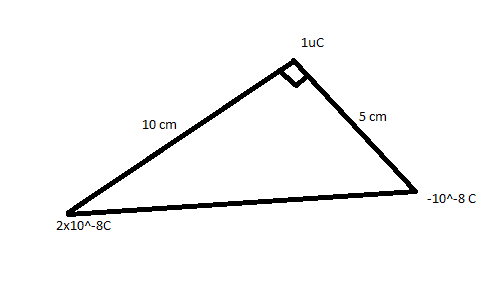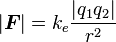# Find force on charge.

## Homework Statement

Find Force at 1uC charge## Homework Equations## The Attempt at a Solution

Assume 1uC charge is at point P 2*10^-8C is at A and 10^-8C is at B
FP = FPA + FPB

FPA = K*2*0.01*1/100
= 0.0002*K
= 2k* 10 ^-4

FPA = K*0.01*1/25
= 0.00004*K
= 4K x 10^-4

sqrt((4K*10^-4)^2 + (2K*10^-4)^2 + 0) --(cos(90) will be 0)
sqrt((20K*10^-16)